Courses

# Test: Transactions & Concurrency Control- 2

## 15 Questions MCQ Test Question Bank for GATE Computer Science Engineering | Test: Transactions & Concurrency Control- 2

Description
This mock test of Test: Transactions & Concurrency Control- 2 for Computer Science Engineering (CSE) helps you for every Computer Science Engineering (CSE) entrance exam. This contains 15 Multiple Choice Questions for Computer Science Engineering (CSE) Test: Transactions & Concurrency Control- 2 (mcq) to study with solutions a complete question bank. The solved questions answers in this Test: Transactions & Concurrency Control- 2 quiz give you a good mix of easy questions and tough questions. Computer Science Engineering (CSE) students definitely take this Test: Transactions & Concurrency Control- 2 exercise for a better result in the exam. You can find other Test: Transactions & Concurrency Control- 2 extra questions, long questions & short questions for Computer Science Engineering (CSE) on EduRev as well by searching above.
QUESTION: 1

### What is the goal of concurrency control protocol?

Solution:

The goal of concurrency control protocol is schedule should be serializable.

QUESTION: 2

### Which of the following concurrency control protocol ensures both conflict serializability and freedom from deadlock? (i) 2 phase locking (ii) Time stamp ordering

Solution:

Time stamp ordering ensure both conflict serializability and free from deadlock.

QUESTION: 3

### If the precedency graph of a given schedule is acyclic, then schedule

Solution:

If the precedency graph of a given schedules is acyclis, then schedule will always be serializable.

QUESTION: 4

When n transactions are run concurrently and in an interleaved manner, the number of possible schedules are_________

Solution:

When ‘n- transactions are runs concurrently then lower that n! interleaved schedules are possible.

QUESTION: 5

​In an DBMS without concurrency control, what consistency problem does the following transaction schedule depict?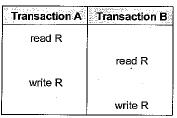Solution:

For the given schedule transaction B reads a value R and is modified by Transaction A which one turn is again modified by Transaction B. In later time if Transaction B failed then the data modified due to transaction A is not recovered hence updation made by A is not matching with the recovered schedule.
Hence, lost update problem occurs.

QUESTION: 6

Consider the following log sequence of two transactions on a bank account, with initial balance 12000, that transfer 2000 to a mortage payment and, then apply a 5% interest.
1. T1 start
2. T1 B old = 12000 new = 10000
3. T1 M old = 0 new = 2000
4. T1 commit
5. T2 start
6. T2 Bold = 10000 new = 10500
​7. T2 commit

Suppose the database system crashed just before log record 7 is written. When the system is restarted, which one statement is true of the recovery procedure?

Solution:

Given that the database system crashed just before log record 7 is written.
Since, before 7 T2 is not committed and T1, has committed. Therefore, T1, need not be redo log records 2 and 3.

QUESTION: 7

​Consider the following schedules involving two transactions. Which one of the following statements is TRUE?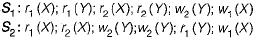Solution: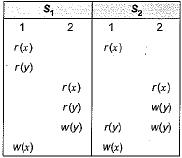Making the dependency graph for S1 and S2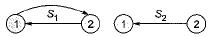According to the above graph we can easily see that
S1 is not conflict serializable but S2 is conflict serializable.

QUESTION: 8

​From transaction scenario given the precedence graph, which of the following is true?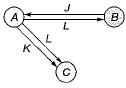Solution:

Since cycle is present in given precedence graph. So transaction schedule is unserializable.

QUESTION: 9

Suppose that process P has been running for several days when a new process Q starts up and begins contending with Pfor resources. Which of the following is true?

Solution:

Process P has been running for several days, when a new process Q starts-up and begins contending with P for resource.

QUESTION: 10

For the schedule given below, which of the following is correct?
3 Write A
5 Write A
6 Write B
8 Write B

Solution:

Initial read of A is done by T1, whereas final write of B is also done by T1. Therefore W1(A), R2(A) and W2(B), R1 (B) are conflicting pairs. The schedule is neither T1 → T2 nor T2 →T1 serializable. Since schedule is not serializable, it can’t occur in a scheme using 2PL protocol.

QUESTION: 11

Consider three data items D1, D2, and D3, and the following execution schedule of transactions T1, T2 and T3. In the diagram, R(D) and W(D) denote the actions reading and writing the data item D respectively.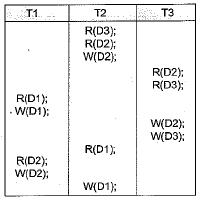Solution:

We have sequence W2(D2)→ W1(D1). Which means T2 →T1 whereas we also have W1(D1) →R2(D1) which means T1→ T2. Both are not possible. Therefore, schedule is not serializable.

QUESTION: 12

​Consider the following schedules involving two transactions. Which one of the following statements is TRUE?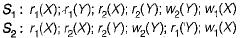Solution:

Consider the table for transaction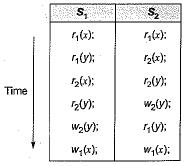There is no serializable conflict in transaction S1 but for statement w1(x) ; there is a conflict in S2 but it is also serializable.

QUESTION: 13

An index is clustered, if

Solution:

Clustered index sort the data in the table based on their key values of the column on which clustered index is created.

QUESTION: 14

Consider two transactions T1 and T2, and four schedules S1 S2, S3, S4 of T1 and T2 as given below: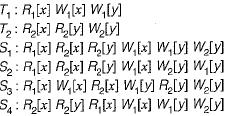Which of the above schedules are conflict- serializable?

Solution:

Schedule S2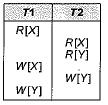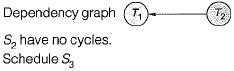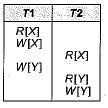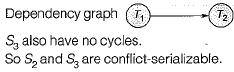QUESTION: 15

Consider the following four schedules due to three transactions (indicated by the subscript) using read and write on a data item x, denoted by r(x) and w(x) respectively. Which one of them is conflict serializable?

Solution:

r1(x); r2(x); w1(x); r3(x); w2(x)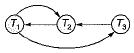Contains cycle; Not conflict serializable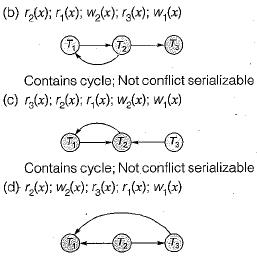Not contains cycle; conflict serializable.